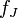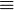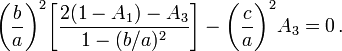# EFE Algorithms

These algorithms that I have stored under the directory, …/fortran/FreeEnergy/EFE

 7 July 2016 jRoot5.for --- When Christodoulou et al. (1995, paper I) generated Figure 3, they picked a value of the total angular momentum that corresponded to a Maclaurin spheroid with eccentricity, e = 0.85. When I made a movie of the free-energy surface that corresponds to 15 (or so) different values of the angular momentum, I decided to adopt values that correspond to Maclaurin spheroids having various values of "e", in steps of 0.005, ending with e = 0.85. Then I decided that I also wanted to use force-balance techniques to tell me precisely what (b/a,c/a) pairs correspond to each of these angular momentum values; but this is not straightforward because, if you are specifying "L", then the pair of axis ratios can only be determined by simultaneously satisfying two nontrivial equations. The "jRoot5.for" routine uses a pair of nested Newton-Raphson loops to identify these axis ratios simultaneously. It begins by specifying 28 different values of "e" (for Maclaurin spheroids), calculating the corresponding "L", then finding the simultaneous roots to obtain (b/a,c/a)_Jacobi with this value of "L". The resulting table of raw numbers can be found at the bottom of: http://www.vistrails.org/index.php/User:Tohline/ThreeDimensionalConfigurations/EFE_Energies#Conserve_Only_L ==================== 6 July 2016 In the file, deriv5.for --- built from old3/deriv3.for --- we have modified subroutine "fJ" to include as well a determination of "fL" and its derivative wrt bovera, namely, "derivL". Definitions of "fL" and "derivL" can be found: http://www.vistrails.org/index.php/User:Tohline/ThreeDimensionalConfigurations/JacobiEllipsoids#Angular_Momentum_Constraint The main routine in this file loops through 20+ axis-ratio pairs and calculates fJ, deriv, fL, & derivL for all pairs. Then, for one pairing (only) it computes the numerical derivative of fL and compares it with the analytic value "derivL". Now, let's plug this generalized subroutine into the "root" routine that uses Newton Raphson technique. ==================== 4 July 2016 jRoot3.for (see jRoot2.for, below) not only lists pairs of axis ratios, but also other useful corresponding quantities such as: A1, A2, A3, omega2, and 5L/M. ==================== 28 June 2016 Circulation7.for -- This generates excellent match to Fig.3 of Christodoulou et al. (1995, paper I). The initial output, "outCirc16.vtr" can be read into VisTrails tool. ==================== 27 June (evening) 2016 Circulation3.for --- This fortran program produces an (b/a,c/a) array of Free-Energy values, then, after normalization, writes both a "cell" and "point" array out in XML format such that it is readable from VisTrails. It needs to be linked only to the doubleELib.o library. ==================== 27 June 2016 File "jRoot2.for" calculates pairs of axis ratios that define the Jacobi ellipsoid equilibrium sequence. gfortran -o exroot jRoot2.o doubleELib.o PROGRAM testnewt: Loops through a group of 'c/a' axis ratios, and for each one, relies upon FUNCTION rtnewt to return the "root", which here is the matching value of 'b/a'. In turn, the function, rtnewt, calls the SUBROUTINE fJ, which provides "rtnewt" with the definition of the governing relation for Jacobi ellipsoids, as well as the analytic definition of the first derivative of this function, with respect to 'b/a'. The definitions of fJ and d(fJ)/dx are provided: http://www.vistrails.org/index.php/User:Tohline/ThreeDimensionalConfigurations/JacobiEllipsoids#Roots_of_the_Governing_Relation FUNCTION rtnewt originated as a function drawn from the Numerical Recipes collection of f77-formatted root-finding algorithms; it uses the Newton-Raphson technique. I modified the original rtnewt in order to perform double-precision arithmetic. The arguments of FUNCTION rtnewt are ... -- covera = c/a; -- x1 and x2 define the bracketed region of b/a within which the root should be found (if not, a message is written); -- xacc = desired precision of root = b/a =================== 25 June 2016 Chandrasekhar provides two relations that define the equilibrium properties of Jacobi ellipsoids. One defines the relationship between the pair of axis ratios (b/a,c/a); the other provides an expression for the corresponding rotation frequency (squared), Omega2. Our desire, here, is to develop a tool -- probably Newton-Raphson technique -- that finds the root(s) of the first of these expressions. Such an iteration technique will require evaluation of the function as well as evaluation of its first derivative. So far, we have developed and debugged a subroutine (fJ) that evaluates the analytic expression for fJ; it also evaluates the first derivative of, fJprime, with respect to (b/a) while holding (c/a) fixed. DOUBLE PRECISION ROUTINES: File fJ.for contains the subroutine (fJ) that evaluates the function and its derivative. In addition, jacobi7.for is a main program that calls this subroutine for 25 different axis-ratio pairs that define the Jacobi sequence (according to EFE); Subroutine fJ is included as part of this jacobi7.for file. gfortran -c jacobi7.for -ffree-form gfortran -o exec jacobi7.o doubleELib.o ./exec > output For supplementary information: http://www.vistrails.org/index.php/User:Tohline/ThreeDimensionalConfigurations/JacobiEllipsoids#Roots_of_the_Governing_Relation 

## Circulation8 (9 July 2016)

Related to our discussion of Free-Energy Surfaces of the c/a versus b/a "EFE Diagram."

## jRoot5 (7 July 2016)

When Christodoulou et al. (1995, paper I) generated Figure 3, they picked a value of the total angular momentum that corresponded to a Maclaurin spheroid with eccentricity, e = 0.85. When I made a movie of the free-energy surface that corresponds to 15 (or so) different values of the angular momentum, I decided to adopt values that correspond to Maclaurin spheroids having various values of "e", in steps of 0.005, ending with e = 0.85. Then I decided that I also wanted to use force-balance techniques to tell me precisely what (b/a,c/a) pairs correspond to each of these angular momentum values; but this is not straightforward because, if you are specifying "L", then the pair of axis ratios can only be determined by simultaneously satisfying two nontrivial equations.

The "jRoot5.for" routine uses a pair of nested Newton-Raphson loops to identify these axis ratios simultaneously. It begins by specifying 28 different values of "e" (for Maclaurin spheroids), calculating the corresponding "L", then finding the simultaneous roots to obtain (b/a,c/a)_Jacobi with this value of "L". The resulting table of raw numbers can be found at the bottom of our discussion titled, "Conserve Only L".

## jRoot2 (27 June 2016)

File "jRoot2.for" calculates pairs of axis ratios that define the Jacobi ellipsoid equilibrium sequence.

gfortran -o exroot jRoot2.o doubleELib.o

PROGRAM testnewt: Loops through a group of 'c/a' axis ratios, and for each one, relies upon FUNCTION rtnewt to return the "root", which here is the matching value of 'b/a'. In turn, the function, rtnewt, calls the SUBROUTINE fJ, which provides "rtnewt" with the definition of the governing relation for Jacobi ellipsoids, as well as the analytic definition of the first derivative of this function, with respect to 'b/a'. The definitions of fJ and d(fJ)/dx are provided in our accompanying discussion titled, "Roots of the Governing Relation".

FUNCTION rtnewt originated as a function drawn from the Numerical Recipes collection of f77-formatted root-finding algorithms; it uses the Newton-Raphson technique. I modified the original rtnewt in order to perform double-precision arithmetic. The arguments of this FUNCTION are ...

• covera = c/a;
• x1 and x2 define the bracketed region of b/a within which the root should be found (if not, a message is written);
• xacc = desired precision of root = b/a

## jacobi7 (25 June 2016)

Chandrasekhar provides two relations that define the equilibrium properties of Jacobi ellipsoids. One defines the relationship between the pair of axis ratios (b/a,c/a); the other provides an expression for the corresponding rotation frequency (squared), Ω2. These are detailed in our accompanying discussion of Jacobi Ellipsoids. Our desire, here, is to develop a tool — probably employing a Newton-Raphson technique — that finds the root(s) of the first of these expressions, namely,$~f_J$$~\equiv$$~\biggl(\frac{b}{a}\biggr)^2 \biggl[ \frac{2(1-A_1)-A_3}{1 - (b/a)^2} \biggr]-\biggl(\frac{c}{a}\biggr)^2 A_3 =0 \, .$

Such an iteration technique will require evaluation of the function as well as evaluation of its first derivative.

So far, we have developed and debugged a subroutine (fJ) that evaluates the analytic expression for fJ; it also evaluates the first derivative of, fJprime, with respect to (b/a) while holding (c/a) fixed.

DOUBLE PRECISION ROUTINES: File fJ.for contains the subroutine (fJ) that evaluates the function and its derivative. In addition, jacobi7.for is a main program that calls this subroutine for 25 different axis-ratio pairs that define the Jacobi sequence (according to EFE); Subroutine fJ is included as part of this jacobi7.for file.

• gfortran -c jacobi7.for -ffree-form
• gfortran -o exec jacobi7.o doubleELib.o
• ./exec > output

For supplementary information, read our accompanying discussion titled, "Roots of the Governing Relation."